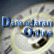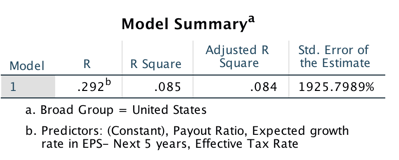##Debt Ratio Regression: January 2017

Variables used in the regression

1. Debt Ratio = Debt/ (Market Value of Equity + Debt): If you can get market value of debt, use it. Else, use book value of debt.
2. Pre-tax ROC = EBIT/ (Book Debt + Book Equity - Cash)
3. Expected growth rate in revenues - next 2 years= You can use expected or even historical earnings growth, if you don't have a revenue growth forecast
4. EBITDA/ Enterprise Value = EBITDA/ (Market Value of Equity + Book value of Debt - Cash)

US Regression## Regression

Market Debt Ratio = 0.2538 + 0.144 (Tax Rate) - .5090 (Expected growth rate) + 0.0181 (Payout Ratio)

With the following t statistics

T statistic for intercept = 19.99

T statsitic for tax rate = 3.86

T statistic for expected growth rate = 11.24

T statistic for payout ratio = 2.02

Global Regression## Regression

Market Debt Ratio = 0.2617 + 0.116 (Tax Rate) - .2314 (Expected growth rate) + 0.0219 (Payout Ratio)

With the following t statistics

T statistic for intercept = 33.28

T statsitic for tax rate = 4.57

T statistic for expected growth rate = 12.30

T statistic for payout ratio = 3.70

• How do I use this regression?

Assume that you want to estimate the market debt ratio for a firm with the following characteristics, using the US regression

Payout Ratio = 40%

Effective Tax Rate= 20%

Expected growth rate in EPS = 15%

• Predicted Value

Expected Debt Ratio= 0.2538 -.144 (20) +.509 (.15) + 0.0181(.40) = ..374 or 37.40%

If your predicted value is less than zero, your predicted debt ratio is zero.